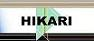| Home | Journals | Books | Paper submission | About us and our mission | News | Contact us |Site Search:

## Online Edition, Vol. 4, 2010, no. 21-24

F. Merovci
Power product inequalities for the \Gamma_k function
Int. Journal of Math. Analysis, Vol. 4, 2010, no. 21-24, 1007-1012.

Zhen-hang Yang
A new proof of inequalities for Gauss compound mean
Int. Journal of Math. Analysis, Vol. 4, 2010, no. 21-24,1013-1018.

A. Abdollahi, E. Rahimi
Multiresolution analysis on the Hardy space of analytic functions
Int. Journal of Math. Analysis, Vol. 4, 2010, no. 21-24, 1019-1025.

S. K. Q. Al-Omari
On the continuous-time wavelet transform of generalized functions
Int. Journal of Math. Analysis, Vol. 4, 2010, no. 21-24, 1027-1034.

Composition operators of class Q*
Int. Journal of Math. Analysis, Vol. 4, 2010, no. 21-24, 1035-1040.

S. Lal, B. P. Dhakal
Approximation of functions belonging to Lipschitz class by triangular matrix method of Fourier series
Int. Journal of Math. Analysis, Vol. 4, 2010, no. 21-24, 1041-1047.

S. K. Datta, E. Jerin
Further results on the common roots of several meromorphic functions and their relative defects
Int. Journal of Math. Analysis, Vol. 4, 2010, no. 21-24, 1049-1054.

A. Rababah
Degree reduction of triangular Bezier surfaces with C^\alpha-vertices
Int. Journal of Math. Analysis, Vol. 4, 2010, no. 21-24, 1055-1066.

Nguyen Dinh Binh
Global attractors for degenerate parabolic equations without uniqueness
Int. Journal of Math. Analysis, Vol. 4, 2010, no. 21-24, 1067-1078.

Shanshan Wang, Yuming Chu
The best bounds of the combination of arithmetic and harmonic means for the Seiffert's mean
Int. Journal of Math. Analysis, Vol. 4, 2010, no. 21-24, 1079-1084.

Yong Yang, Zhi Jie Jiang
Products of differentiation and composition from weighted Bergman spaces to some spaces of analytic functions on the upper half-plane
Int. Journal of Math. Analysis, Vol. 4, 2010, no. 21-24, 1085-1094.

Nguyen Van Luong, Nguyen Xuan Thuan
Common fixed point theorems for weakly compatible maps through generalized altering distance function
Int. Journal of Math. Analysis, Vol. 4, 2010, no. 21-24, 1095-1104.

M. Matar
Controllability of fractional semilinear mixed Volterra-Fredholm integrodifferential equations with nonlocal conditions
Int. Journal of Math. Analysis, Vol. 4, 2010, no. 21-24, 1105-1116.

Dual variational formulation applied to an acoustic model problem
Int. Journal of Math. Analysis, Vol. 4, 2010, no. 21-24, 1117-1133.

A. Kananthai
On the diamond wave operator
Int. Journal of Math. Analysis, Vol. 4, 2010, no. 21-24, 1135-1143.

H. Menken, A. Asan
Recurrent relations for the coefficients of some p-adic differential equations
Int. Journal of Math. Analysis, Vol. 4, 2010, no. 21-24, 1145-1152.

A. Boutoulout, H. Bourray, F.Z. El Alaoui
Regional boundary observability for semi-linear systems approach and simulation
Int. Journal of Math. Analysis, Vol. 4, 2010, no. 21-24, 1153-1173.

S.K.Q. Al-Omari
On the generalized Struve transformation
Int. Journal of Math. Analysis, Vol. 4, 2010, no. 21-24, 1175-1180.

H. S. Mehta, R. D. Mehta
Weak (i) - peak sets for a real function algebra
Int. Journal of Math. Analysis, Vol. 4, 2010, no. 21-24, 1181-1188.